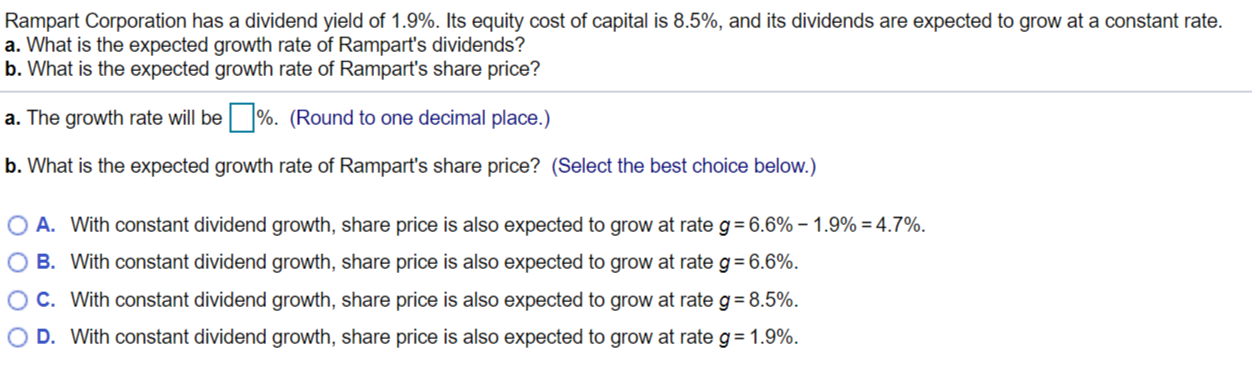### Create an Account

Home / Questions / Rampart Corporation has a dividend yield of 1.9%. Its equity cost of capital is 8

# Rampart Corporation has a dividend yield of 1.9%. Its equity cost of capital is 8Rampart Corporation has a dividend yield of 1.9%. Its equity cost of capital is 8.5%, and its dividends are expected to grow at a constant rate. a. What is the expected growth rate of Rampart&#39;s dividends? b. What is the expected growth rate of Rampart&#39;s share price? a. The growth rate will be %. (Round to one decimal place.) b. What is the expected growth rate of Rampart&#39;s share price? (Select the best choice below.) O A. With constant dividend growth, share price is also expected to grow at rate g = 6.6% -1.9% = 4.7%. O B. With constant dividend growth, share price is also expected to grow at rate g=6.6%. O C. With constant dividend growth, share price is also expected to grow at rate g = 8.5%. D. With constant dividend growth, share price is also expected to grow at rate g= 1.9%. O

Jan 31 2020 View more View LessGet Solution